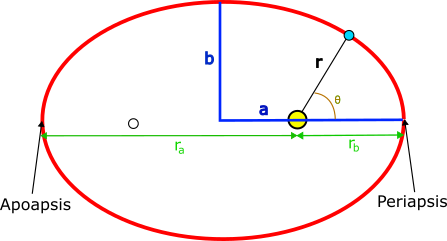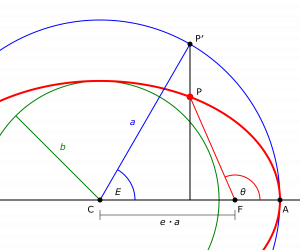# Introduction of the six basic parameters describing satellite orbits

A satellite orbit is always in a plane around the heaviest body. The plane contains this body’s center. In this article, we will discuss the six classical orbital elements.

## The Orbital Elements

This system has six degrees of freedom. We need six parameters to describe the orbit. Three of the parameters we will use, describe how the plane looks like and the position of the satellite on the ellipse, and the other three parameters will describe how that plane is oriented in the celestial inertial reference frame and where the satellite is in that plane. These six parameters are called the Keplerian elements or orbital elements.Figure 1: Definition of semi-major axis a, semi-minor axis b, true anomaly θ, apoapsis, periapsis and their radius ra and rb. Illustrated by Andøya Space Education.

## Semi-Major and Semi-Minor Axis

The general elliptical orbit of a planet orbiting its closest star is seen in figure 1. The heaviest body in the Keplerian orbit (here seen as a star), is in one of the focal points of the orbiting satellite (the planet). The other focal point is empty and is marked with a black dot. The shortest radius in the ellipse is the semi-minor axisand the largest radius is the semi-major axis. Both distances are measured from the center of the ellipse. The semi-major axisis the first Keplerian parameter, which we will use to describe the size of the ellipse.

In the case of man-made satellites, it is often practical to relate the parameters to what we measure from Earth, and not some arbitrary empty point in space. Generally, the point where the satellite is the closest to the central body is called the periapsis, with the length to the central body usually denoted as. The point where the satellite is the farthest away is called the apoapsis, and has the associated length. The semi-major axis is on the line segment between the periapsis and the apoapsis, and it is half of the distance between them, that is.

## Eccentricity

We have now given a parameter of the size of the orbit, but to completely define the orbit we also need to know it’s shape. This is given by the second orbital parameter, the eccentricity. It can be defined fromand, and is quite simple to calculate. For an elliptical orbit it is given asIt does not matter what units the two radii are given in as the eccentricity is unitless. As an example, what is the eccentricity of a circle? Since a circle has constant radius, we must have thatmaking the eccentricity. An ellipse will have an eccentricity fromup to (but not including). What kind of orbits we get for even higher eccentricities, we will come back to shortly. Ellipses with different eccentricities are shown in figure 2.Figure 2: Eccentricities in orbits where one of the focal points is fixed, as well as the periapsis radius. Eccentricities from 0 to 0.9 are shown to increase in steps of 0.1. Note that neither the semi-major axis a nor the semi-minor axis b is constant here. Illustrated by Andøya Space Education.

In figure 1 we can see that the radius r is given from the central body to the center of the satellite. The angleis the angle between the semi-major axis and the line between the central body to the satellite and varies in time when the satellite orbits the central body. When the satellite is at perigee the angle isand when it is at apogee the angle is. The angleis most often called the true anomaly and is the third orbital parameter. It describes the orbital position of the satellite at any specific time.

Now we have found the first three parameters describing the orbit and the satellite position in it. The following expressiondescribes the orbit in polar coordinates as a function of the true anomaly. We stated previously that ellipses have eccentricities from 0 up to 1, and for these cases the radius will be well defined for all anglesas can easily be seen.Figure 3: Definition of the true and eccentric anomalies. Illustration derived from a derived work by the Wikimedia-user CheChe (original by Brews ohare). Licenced under CC BY-SA 4.0.

The true anomaly is one of the three anomalies/parameters describing the position around the orbit. The other two anomalies are called the eccentric anomaly and mean anomaly, and they are used to relate the position of the satellite (from the true anomaly) to the time since the passing of the perigee. The eccentric anomalyis an actual angle shown in figure 3. The position of the satellite is at the point P with true anomaly. The blue (largest) circular orbit has a constant radius equal to the semi-major axisof the satellite orbit (shown in red in the figure). The eccentric anomaly is the left anglein the triangle CQP’, where the line segment QP’ is the line segment perpendicular to the periapsis–apoapsis line through the point P (the actual position of the satellite) to the point P’, which is the intersection with the circular blue orbit (the point Q is not marked on the figure). This makes the length of the hypotenuse (the line segment CP’) the satellite semi-major axis. The relation between the true anomalyand the eccentric anomaly isThe mean anomalyis not a true angle. It is defined aswhereis the time since the last crossing of periapsis and, whereis the orbital period of the satellite orbit. The mean anomaly is an angle an imaginary satellite would have if it where in a circular arbit around the point C (figure 3) with a time periodequal to the true satellite orbit. The reason of why we define the eccentric and mean anomaly is to find the relationship between the true anomaly and the time since periapsis. The relationship between the mean and eccentric anomaly iswhereis the eccentricity of the (real) satellite orbit. This equation is a transcendental equation that cannot be solved analytically forbut several resources online can help with solving equations like this, e.g. WolframAlpha.com. Knowing the true anomaly, the time since periapsis can be found by first calculating the eccentric anomaly and then the mean anomaly. Knowing the time since periapsis, however, the mean anomaly is first calculated, but finding the eccentric anomaly need to be found numerically. That solution is then used to find the true anomaly.

## Non-eliptic orbits

Orbits withare called parabolas, while orbits with higher eccentricity (i.e.) are called hyperbolas. These orbits have an infinitely long orbital period—they never return to a point they have already been at, because they have a higher velocity than the escape velocity (parabolas actually have exactly the escape velocity). Some comets observed from Earth travel along such orbits, but satellites very seldomly do. These are thus special cases, which we will not discuss further.

## Parameters used to describe the orientation in space

We use the celestial reference frame to define some additional parameters. See figure 5. The angle between the elliptical plane and the reference plane is called the inclination, and is measured from the reference plane to the orbital plane at the ascending node. The inclination is the first orbital parameter which describes the orientation of the orbit. The second parameteris the argument of periapsis, also called the argument of perigee, and is the angle between the ascending node and the semi-major axis of the ellipse. The last orbital parameter is the longitude of ascending node, which is the angle between the reference direction and the ascending node in the travelling direction of the satellite from the ascending node (counter-clockwise as seen in the figure).

The six orbital parameters are shown in the video below:

A closer description of the orbital parameters is given in this video, but note that a different base angle is used in the true anomaly:

## Finding orbital parameters from observation

It is impractical to have to wait until the satellite reaches its apogee and perigee to measure it, and we instead want a method where we can find the orbital parameters from observations at different, arbitrary orbital positions.

There are numerous ways of doing this. We will follow the so-called Gibbs method. This method takes three different positions along the orbit, and for one of those positions it calculates the velocity. From three-dimensional position and velocity vectors at a given time one can calculate all the orbital parameters discussed above. The method will here be given as an algorithm without explaining the background. The method is described well in the literature. Curtis’ Orbital Mechanics for Engineering Students (2010), for example, gives an excellent introduction to this and other techniques.

We have three position vectorsandalong the orbit.

1. Find the absolute value of the three vectors (i.e. the distance from the focal point to the satellite),.
2. Calculate, whereis the cross product.
3. Calculate.
4. Calculate.
5. The velocity in the positionis then, whereis called the gravitational parameter. For Earth,.

Note that there is a difference between a vector (with an arrow) and the length of the vector. The vectorsandcombined are called the state vector. From this, we can calculate all the different parameters expanding on that algorithm:

1. First find the tangential velocity component by.
2. Then find the eccentricity vector. The length ofis the well-known eccentricity.
3. The true anomalyatis given by, assuming the radial velocity is positive (including 0). If the radial velocity is less than zero, replacewith.
4. From the equation of motional energy (introduced later in this chapter), we find the semi-major axis by.
5. For reference we also find the three parameters describing the plane orientation. First find the specific angular momentum. Then find the vectorwhereis the unit vector in z direction.
6. The inclinationis calculated from.
7. The right ascension of the ascending node (RAAN) / longitude of the ascending node,, is calculated from, provided that. If, replacewith. For non-inclined orbits (where), the RAAN is conventionally set to.
8. For non-zero inclinations, the argument of perigee is calculated from. When the inclination is zero, the argument of perigee is found using, sincewhen.